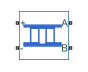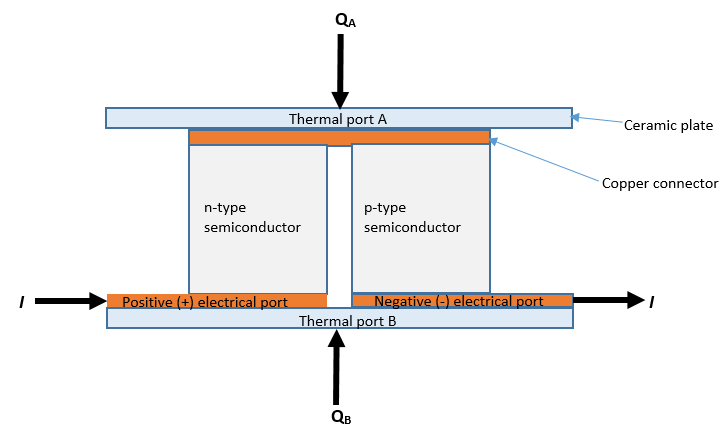# Peltier Device

Electrothermal converter

•Libraries:
Simscape / Electrical / Sensors & Transducers

## Description

The Peltier Device block represents a converter between the electrical and thermal energy:

• With no current flowing, if the temperature presented at thermal port B is greater than the temperature presented at thermal port A, then there is a positive potential difference measured from the positive to the negative electrical port.

• When the block acts as a cooling device, a positive current causes heat to flow from port A to port B, cooling port A relative to port B.The defining equations are:

`$\begin{array}{l}{Q}_{A}=\alpha {T}_{A}I-\frac{1}{2}{I}^{2}R+K\left({T}_{A}-{T}_{B}\right)\\ {Q}_{B}=-\alpha {T}_{B}I-\frac{1}{2}{I}^{2}R+K\left({T}_{B}-{T}_{A}\right)\\ W=VI\\ W+{Q}_{A}+{Q}_{B}=0\end{array}$`

where:

• QA is heat flow into port A.

• QB is heat flow into port B.

• TA is port A temperature.

• TB is port B temperature.

• W is electrical power (positive when flowing into the block).

• V is potential difference across the + and - ports.

• I is electrical current, positive from + to - port.

• R is total electrical resistance.

• α is Seebeck coefficient.

• K is thermal conductance.

Substituting for powers and dividing all terms by current gives the electrical equation:

`$V=\alpha \left({T}_{B}-{T}_{A}\right)+IR$`

The block has a logging variable `power_dissipated` (Dissipated power). This variable reports the DC electrical power, that is, the average electrical power over one AC cycle if you drive the device with an AC source. In terms of equations it is equal to the instantaneous value of I2R and is useful in a cooling application to indicate the nonproductive portion of the heat flow. In a heating application, the interpretation of dissipated power is less useful.

## Ports

### Conserving

expand all

Electrical conserving port associated with the positive terminal.

Electrical conserving port associated with the negative terminal.

Thermal conserving port. When the block acts as a cooling device, a positive current causes heat to flow from port A to port B, cooling port A relative to port B.

Thermal conserving port.

## Parameters

expand all

### Electrical

Measure of the magnitude of an induced thermoelectric voltage in response to a temperature difference across that material.

Total electrical resistance between the + and - ports.

### Thermal

Thermal conductance between the A and B ports.

## Version History

Introduced in R2018b